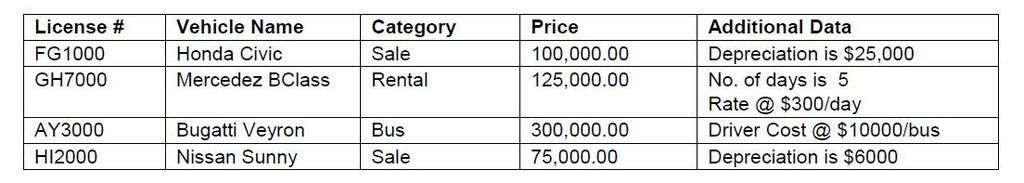Vehicle Class using JavaPosted by Samath13719 March 19, 2015

COMP1161 Motors both sells and rents vehicles. It rents two types of vehicles – Bus and Car.
Every vehicle has a license number, a make , a model and price.

Write the class Vehicle to represent a motor vehicle. Your code shall include:
- Declarations for all attributes that belong in this class. These will be those attributes that all vehicles have.
- A constructor that gets (as parameters) a vehicle’s number, a make, a model and price (in that order).
- An accessor method named getVehicleName that returns the vehicle’s name in the form make, model (e.g. “Suzuki Swift”)
- A method named price that returns the price of the vehicle.
- A toString method which returns a string in the following format:

Vehicle Name: Make Model

Example:

Vehicle Name : Suzuki Swift
Price : \$200, 000.00

Write the class Sale as a subclass of Vehicle. Your code should include:
- Declarations of all attributes that vehicle on sale has which are not already declared in the parent class.

- A constructor that accepts (as parameters) a vehicle’s number, a make, a model, price and depreciation value (in that order).
- A method called setDepreciation that accepts the percentage rate, calculates the depreciation value (which is rate * price of vehicle) and set this attribute on the object.
- A toString method that formats its returned value in the following manner:

Vehicle Name : Suzuki Swift
Price : \$200, 000.00
Depreciation : \$ 50,000.00
Selling Price : \$150,000.00

Write the class Rental to represent a vehicle to be rented. This class is also a subclass of the Vehicle class. Your code should include:
- Declarations of all attributes that a rental vehicle has which are not already declared in the parent class.
- A constructor that accepts a vehicle’s number, a make, a model, price, number of days for rental and the rate per day.
- The rental price of the vehicle is calculated as number of days times the rate.
- A toString method that formats its returned value in the following manner:

Vehicle Name : Suzuki Swift
Price : \$200,000.00
Rental Info:
Number of days : 5
Rate per day : \$ 2,000.00
Total : \$10,000.00

Write the class Bus to represent a bus on rental. Your code should include:
- Declarations of all attributes that a rental bus has which are not already declared in the parent class.
- A constructor that a vehicle’s number, a make, a model, price, number of days for rental, the rate per day and driver cost.
- A method named setDriverCost which accepts the driver’s cost and sets this value on the object.
- A toString method that formats its returned value in the following manner:

Vehicle Name : Suzuki Swift
Price : \$200,000.00
Rental Info:
Number of days : 5
Rate per day : \$ 2,000.00
Total : \$10,000.00
Driver cost : \$12,000.00
Final cost : \$22,000.00

Override the price method in each subclass so that price is calculated correctly for each type of vehicle.

1. Write a driver class with a main method which creates an array with the data from the following table and displays the array contents.2. Modify your code to use the setDepreciation method to set the depreciation value for the element at position 0 using the rate 20%.
3. Modify your code to use the setDriverCost method to set the cost for driver for the element at position 2 to \$15,000.
4. Display the contents of the array.

Vehicle Class:

```public class Vehicle
{
protected String make;
protected String model;
protected double price;

public Vehicle(String l_n, String v_make, String v_model, double v_price)
{
make = v_make;
model = v_model;
price = v_price;
}

public String getVehicleName()
{
return make+" "+model;
}

public double price()
{
return price;
}

public String toString()
{
String result;

result = "License # : "+ license_number+ "\nVehicle Name : "+getVehicleName()+"\nPrice : "+price;
return result;
}
}
```

Rental Class:

```public class Rental extends Vehicle
{
protected int number_of_days_for_rental;
protected double rate_per_day;

public Rental(String l_n, String v_make, String v_model, double v_price, int no_of_day, double r_p_d)
{
super(l_n,v_make,v_model,v_price);
number_of_days_for_rental = no_of_day;
rate_per_day = r_p_d;
}

public double price()
{
return number_of_days_for_rental*rate_per_day;
}

public String toString()
{
String result;
result = super.toString();

result += "\nRetal Info: "+"\n\tNumber of Days: "+number_of_days_for_rental+"\n\tRate per day: "+rate_per_day+"\n\tTotal: "+price();
return result;
}
}
```

Bus Class:

```public class Bus extends Rental
{
private double driver_cost;

public Bus(String l_n, String v_make, String v_model, double v_price, int no_of_day, double r_p_d, double dri_cost)
{
super(l_n,v_make,v_model,v_price,no_of_day, r_p_d);
driver_cost = dri_cost;
}

public void setDriverCost(double s_driver)
{
driver_cost = s_driver;
}

public String toString()
{
String result;
double final_cost = driver_cost + super.price();
result = super.toString();

result += "\n\tDriver Cost: "+driver_cost+"\n\tFinal cost: "+final_cost;
return result;
}

public double price()
{
return super.price()+driver_cost;
}
}
```

Sale Class:

```

public class Sale extends Vehicle
{
private double depreciation,sell_price;
private double rate;

public Sale(String vehnum,String make,String model, double p, double depval)
{
super(vehnum,make,model,p);
depreciation = depval;
sell_price = p;
}

public void setDepreciation(double rate)
{
depreciation = (rate/100)*super.price();
}

public double price()
{
sell_price = super.price() - depreciation;
return sell_price;
}

public String toString()
{
return (super.toString() + "\n Depreciation\t:"+ depreciation +"\n Selling Price \t:"+ price());
}

}

```

TestVehicle:

```public class TestVehicle {

public static void main(String[] arg)
{
Vehicle[]comp1161_motors = new Vehicle ;
Sale veh1 = new Sale("FG1000", "Honda", "Civic", 100000.00, 25000);
Rental veh2 = new Rental("GH7000", "Mercedez", "BClass", 125000.00, 5, 300);
Bus veh3 = new Bus("AY3000","Bugatti","Veyron",300000.00, 5, 300,10000);
Sale veh4 = new Sale("HI2000", "Nissan", "Sunny",75000.00,6000.00);

Vehicle me = new Bus("BW1099","Nissan","Tida", 120000, 10, 400, 1000);
comp1161_motors = veh1;
comp1161_motors = veh2;
comp1161_motors = veh3;
comp1161_motors = veh4;

((Sale)comp1161_motors).setDepreciation(20.0);
((Bus)comp1161_motors).setDriverCost(15000.0);

for( Vehicle veh : comp1161_motors)
{
System.out.println();
System.out.println(veh);
}
}

}

```